# 31 Year NEET Previous Year Questions: Semiconductor Electronics - 1

## 25 Questions MCQ Test Topic-wise MCQ Tests for NEET | 31 Year NEET Previous Year Questions: Semiconductor Electronics - 1

Description
Attempt 31 Year NEET Previous Year Questions: Semiconductor Electronics - 1 | 25 questions in 50 minutes | Mock test for NEET preparation | Free important questions MCQ to study Topic-wise MCQ Tests for NEET for NEET Exam | Download free PDF with solutions
QUESTION: 1

### Sodium has body-centered packing. The distance between the two nearest atoms is 3.7 Å. The lattice parameter is: 

Solution: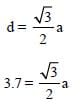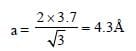QUESTION: 2

### Which one of the following statements is false?    

Solution:

The majority carriers in an n-type semiconductor are electrons.

QUESTION: 3

### Which one of the following bonds produces a solid that reflects light in the visible region and whose electrical conductivity decreases with temperature and has a high melting point?    

Solution:

Metals (metallic bonds) have these physical properties:

• Metals reflect the photons of the visible spectrum (light) falling on their surface, thus metals are often silver-white or greyish with the characteristic specular reflection of metallic lustre.
• The electrical conductivity of metals decreases with a rise in temperature.
• Metals have a high melting point.
QUESTION: 4

The device that can act as a complete electronic circuit is:


Solution:

Integrated circuit can act as a complete
electronic circuit.

QUESTION: 5

A common emitter amplifier has a voltage gain of 50, an input impedance of 100 Ω and an output impedance of 200 Ω. The power gain of the amplifier is:


Solution:

Power gain = Voltage gain * Current gain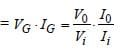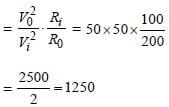QUESTION: 6

To get an output Y = 1 from the circuit shown below, the input must be: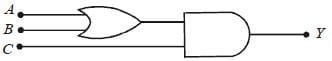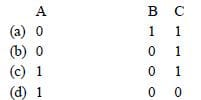Solution: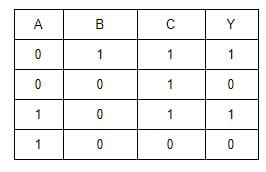For (a) & (c) we get output 1.

QUESTION: 7

For transistor action:
(1) Base, emitter and collector regions should have similar size and doping concentrations.
(2) The base region must be very thin and lightly doped.
(3) The emitter-base junction is forward biased and the base-collector junction is reverse biased.
(4) Both the emitter-base junction as well as the base-collector junction are forward biased.

Solution:
• For transistor action, the base region must be very thin and lightly doped.
• Also, the emitter-base junction is forward biased and the base-collector junction is reverse biased.
QUESTION: 8

The following Figure shows a logic gate circuit with two inputs A and B and the output Y. The voltage waveforms of A, B and Y are given: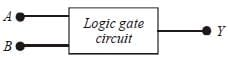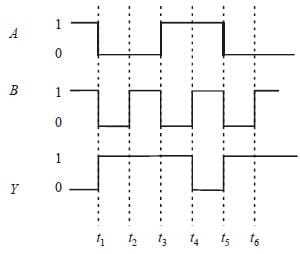The logic gate is:

Solution:

From the given waveforms, the truth table
is as follows: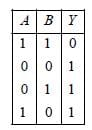The above truth table is for the NAND gate.

QUESTION: 9

A transistor is operated in common emitter configuration at VC = 2V such that a change in the base current from 100 μA to 300 μA produces a change in the collector current from 10 mA to 20 mA. The current gain is:


Solution:

The current gain: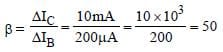QUESTION: 10

In forward biasing of the p–n junction:


Solution:

In forward biasing of the p-n junction, the positive terminal of the battery is connected to the p-side and the negative terminal of the battery is connected to the n-side. The depletion region becomes thin.

QUESTION: 11

Symbolic representation of four logic gate are shown as:   
(i)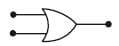(ii)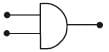(iii)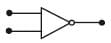(iv)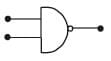Pick out which ones are for AND, NAND and NOT gates, respectively.

Solution: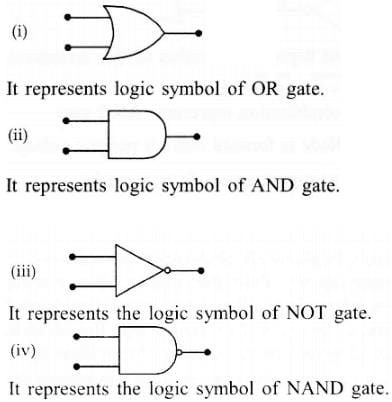QUESTION: 12

If a small amount of antimony is added to germanium crystal then:


Solution:
• When a small amount of antimony (pentavalent) is added to the germanium crystal then the crystal becomes an n-type semiconductor.
• Therefore, there will be more free electrons than holes in the semiconductor.
QUESTION: 13

In the following figure, the diodes which are forward biased are:
[2011M]

(i)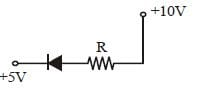(ii)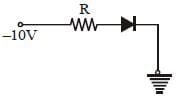(iii)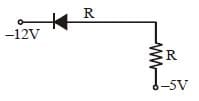(iv)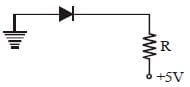Solution:

Only in (A) and (C) diodes are forward biased as p-type should be at higher potential and n-type at lower potential.

QUESTION: 14

Pure Si at 500K has an equal number of electrons (ne) and hole (nh) concentrations of 1.5 × 1016 m–3. Doping by indium increases nto 4.5 × 1022 m–3. The doped semiconductor is of:
[2011M]

Solution: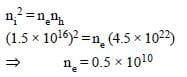ne = 5 × 10& nh = 4.5 × 1022
⇒ nh >> ne
∴ Semiconductor is p-type and ne = 5 * 109 m–3.

QUESTION: 15

A Zener diode, having a breakdown voltage equal to 15V, is used in a voltage regulator circuit shown in the figure. The current through the diode is:
[2011M]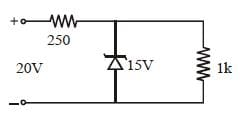Solution:

The voltage across the Zener diode is constant.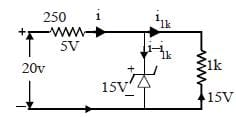Current in 1kΩ resistor = I1kΩ = 15 Volt / 1 kΩ = 15 mA
Current in 250Ω resistor = I250Ω = (20 - 15) / 250 = 5V/250 = 20 mA
Izener diode = (20 - 15) = 5 mA

QUESTION: 16

Two ideal diodes are connected to a battery as shown in the circuit. The current supplied by the battery is: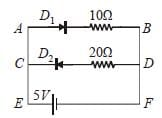Solution:

Here D1 is in forward bias and D2 is in
reverse bias. So, D1 will conduct and D2 will not conduct. Thus, no current will flow through DC.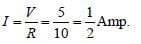QUESTION: 17

In a CE transistor amplifier, the audio signal voltage across the collector resistance of 2kΩ is 2V. If the base resistance is 1kΩ and the current amplification of the transistor is 100, the input signal voltage is:


Solution: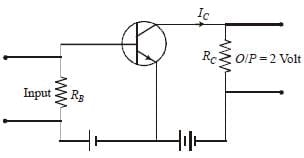The output voltage, across the load RC
V0 = IC RC = 2
Collector current (IC):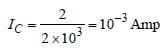Current gain (β):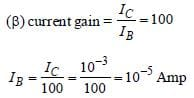Input voltage (Vi):

Vi = IBRB = 10–5 * 103 = 10–2 Volt
Vi = 10 mV

QUESTION: 18

C and Si both have the same lattice structure, having 4 bonding electrons in each. However, C is an insulator whereas Si is an intrinsic semiconductor. This is because:


Solution:

The four bonding electrons in the case of C lie in the second orbit, whereas in the case of Si they lie in the third orbit so loosely bounded valence electrons in Si as compared to C.

QUESTION: 19

Transfer characteristics [output voltage (V0) vs input voltage (V1)] for a base biased transistor in CE configuration is as shown in the figure. For using a transistor as a switch, it is used: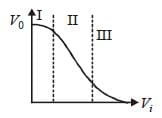Solution:

In the given graph:

• Region (I) Cut-off region
• Region (II) Active region
• Region (III) Saturation region

Transistor as a switch is used in the cut-off or saturation region. So the correct option is B.

QUESTION: 20

The figure shows a logic circuit with two inputs A and B and the output C. The voltage waveforms across A, B and C are given. The logic circuit gate is: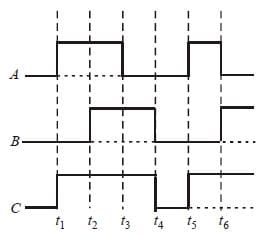Solution: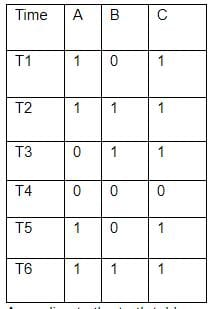According to the truth table, we can clearly see that it is an OR Gate

QUESTION: 21

The input resistance of a silicon transistor is100 Ω. Base current is changed by 40 μA which results in a change in collector current by 2 mA. This transistor is used as a common emitter amplifier with a load resistance of 4 KΩ. The voltage gain of the amplifier is:
[2012M]

Solution: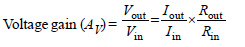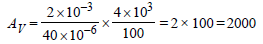QUESTION: 22

To get an output Y = 1 in the given circuit which of the following input will be correct:
[2012M]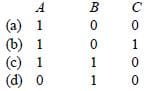Solution:
• C should be always 1 and at least one of A & B should be 1 (as they are connected to OR Gate) to get Y = 1
• Option (b) is the only option where C = 1 and at least one of A & B is 1 (A = 1 & B = 0)
QUESTION: 23

In an n-type semiconductor, which of the following statement is true?


Solution:

In an n-type semiconductor, holes are minority carriers and pentavalent atoms are dopants.

QUESTION: 24

In a common emitter (CE) amplifier having a voltage gain G, the transistor used has transconductance 0.03 mho and a current gain of 25. If the above transistor is replaced with another one with transconductance 0.02 mho and a current gain of 20, the voltage gain will be:


Solution: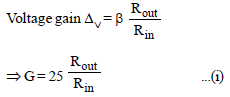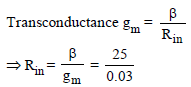Putting this value of Rin in equation (i):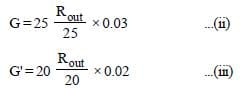From eqs. (ii) and (iii) we get:
Voltage gain of new transistor,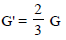QUESTION: 25

The output (X) of the logic circuit shown in figure will be: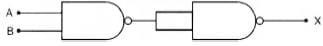Solution: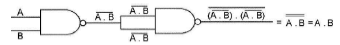Output X = A.B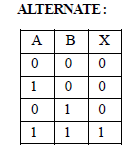Use Code STAYHOME200 and get INR 200 additional OFF Use Coupon Code